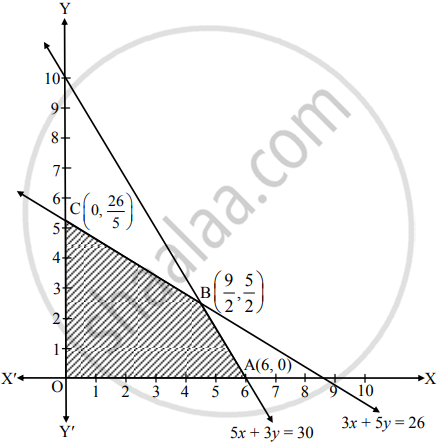# Solve the following L.P.P. by graphical method : Maximize : Z = 7x + 11y subject to 3x + 5y ≤ 26, 5x + 3y ≤ 30, x ≥ 0, y ≥ 0. - Mathematics and Statistics

Graph

Solve the following L.P.P. by graphical method :

Maximize : Z = 7x + 11y subject to 3x + 5y ≤ 26, 5x + 3y ≤ 30, x ≥ 0, y ≥ 0.

#### Solution

The draw the feasible region, construct table as follows:

 Inequality 3x + 5y ≤ 26 5x + 3y ≤ 26 Corresponding equation (of line) 3x + 5y  26 5x + 3y = 30 Intersection of line with X-axis (26/3, 0) (6, 0) Intersection of line withY-axis (0,  26/5) (0, 10) Region Origin side Origin side

Shaded portion OABC is the feasible region,

whose vertices are O(0, 0), A(6, 0), B and C (0,  26/5).

B is the point of intersection of the lines 5x + 3y = 30 and 3x + 5y = 26.
Solving the above equations, we get

x = (9)/(2), y = (5)/(2)

∴ B = (9/2 , 5/2) ≡ (4.5, 2.5)Here, the objective function is Z = 7x + 11y
∴ Z at O(0, 0) = 7(0) + 11(0) = 0
Z at A(6, 0) = 7(6) + 11(0) = 42

Z at B (9/2, 5/2) = 7(9/2) + 11(5/2) = (63 + 55)/(2) = 59

Z at C(0, 26/5) = 7(0) + 11(26/5)  (286)/(5) = 57.2

∴ Z has maximum value 59 at B(9/2, 5/2).
i.e. at B(4.5, 2.5)
∴ Z is maximum, when x = (9)/(2)  "and"  y = (5)/(2)
i.e. when x = 4.5 and y = 2.5

Is there an error in this question or solution?
Chapter 6: Linear Programming - Exercise 6.2 [Page 101]

Share